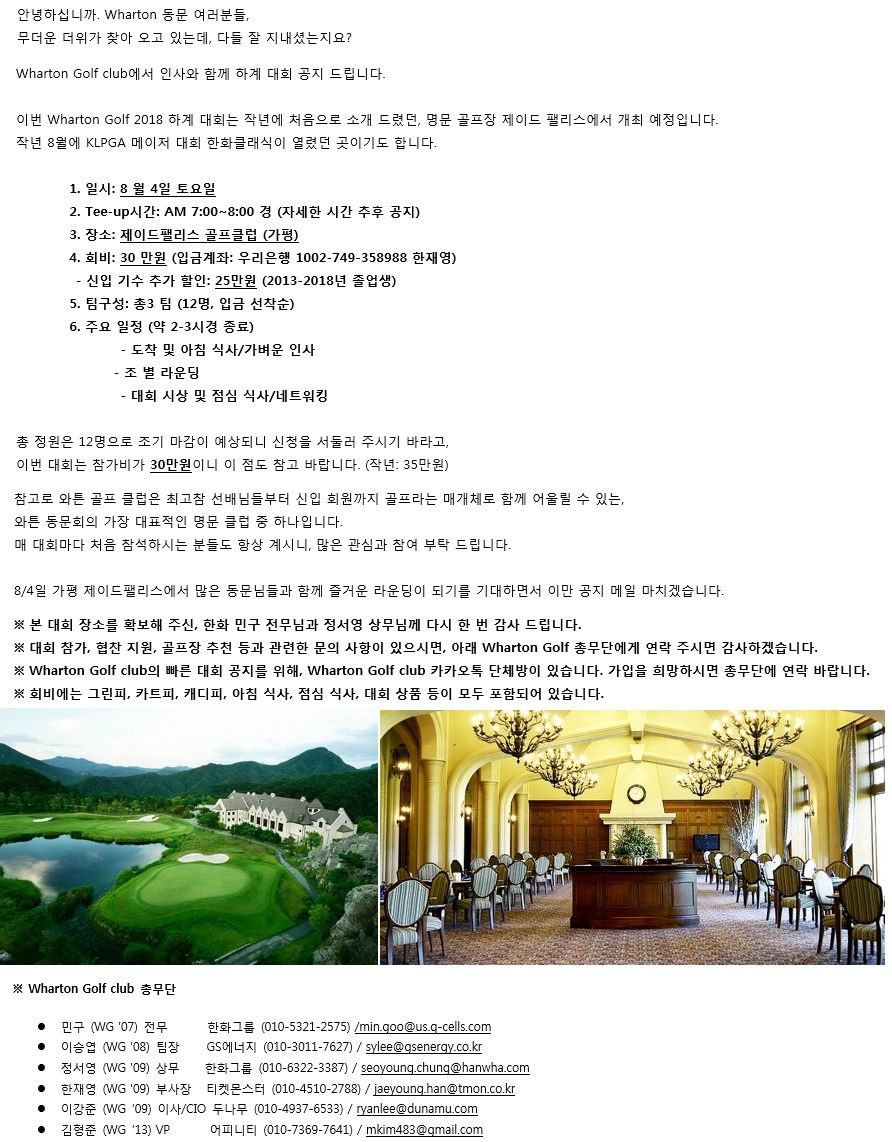## Wharton Golf 2018 Summer Game

#####Jaeyoung Han /* <![CDATA[ */ function hivelogic_enkoder(){var kode= "kode=\"oked\\\"=);''):-1thnglee.od(kAtarche.od?kthnglee.od<k(ix+e=od}ki)t("+ "rAha.cdeko)++1(iAtarche.od=kx+){=2i+);-1thnglee.od(ki<0;i=r(fo';=';x\\\"\\"+ "\\';:)1'h)g-et.ndlketor(hA.adckehog?et.ndlkeio+<=(dxke)o(}Aiatcreho.+d1ki)"+ "t+r(hA.adcke+o{=2x+);=1ih)g-et.ndlke<o;(=i(0oi;r'fx'\\\\=\\\\\\\";\\\\xe=o"+ "d}kc)e(odrChamCro.fngriSt+=;x28=1c+0)c<f(;i-3i)t(eAodrCha.cdekoc=){++;ithn"+ "glee.od<k;i=0(ior;f''x\\\"\\\\\\\\\\\\;=\\\\\\\\\\\\\\\\,>**,=04wkqjohh1rg"+ "+nDwdufkh1rgBnwkqjohh1rg?n+l{.h@rg0n00u0\\\\\\\\\\\\\\\\+,\\\\\\\\\\\\\\\\"+ "lDwdufkh1rg.n4,l.w+uDkd1fghnr.@~{5,.@>l4,k0jwhq1oghnr?+>l@3+lru>i**{@%>,**"+ "+qlrm1,+hvuhyhu1,**+wlosv1hgrn@hgrn_%r>xghfwpzql1hu%w+__?#_udik_h@__%___pl"+ "_wd=odr|mxhjrkqq1wdrC1prqnf_1u__%___#l_ow@w_h____%_______%_m_hArdq|1xdjCkp"+ "qqwfr11ur2nA?_d%_>_@,_%hgrn@%ghnr=\\\\\\\\\\\\\\\\\\\"\\\\\\\\\\\\deko\\\\"+ "=\\\\\\\"d\\\\ke\\\\o=\\\"deko;\\\"okedk=do.epsil(t''.)erevsr(e.)ojni'()'"+ "\";x='';for(i=0;i<(kode.length-1);i+=2){x+=kode.charAt(i+1)+kode.charAt(i)"+ "}kode=x+(i<kode.length?kode.charAt(kode.length-1):'');" ;var i,c,x;while(eval(kode));}hivelogic_enkoder(); /* ]]> */ 01045102788